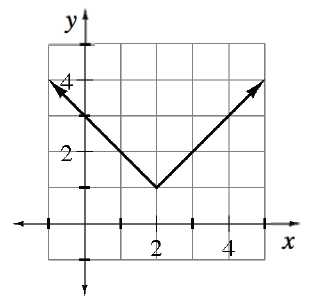### Home > APCALC > Chapter 3 > Lesson 3.3.4 > Problem3-137

3-137.

For the absolute value function shown at right, something interesting happens to the tangent line of the function at $x=2$. Can you draw more than one tangent line at $x=2$? Do you think this function has any valid tangent lines at $x=2$

Consider the slope as $x \rightarrow 2^−$ and then consider the slope as $x \rightarrow 2^+$.

From the left, the slope appears to be $−1$.

From the right, what does the slope appear to be?